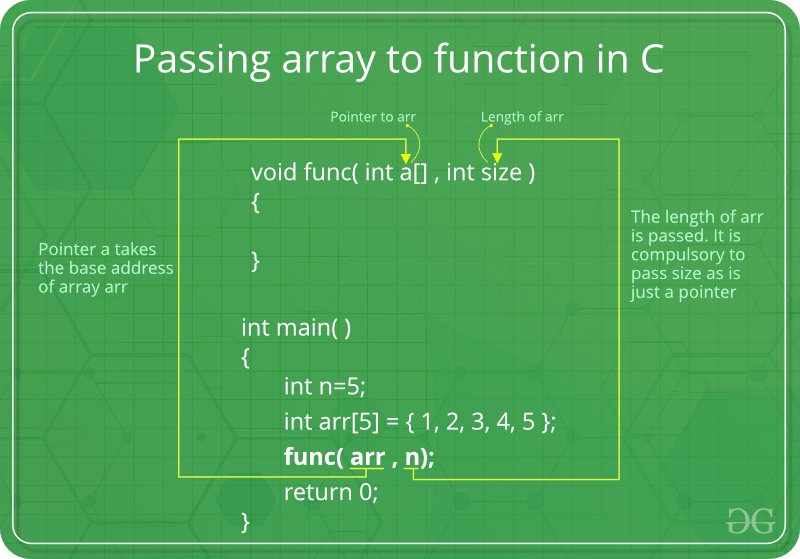Related Articles

# How arrays are passed to functions in C/C++

• Difficulty Level : Medium
• Last Updated : 19 Mar, 2021

In C, when we pass an array to a function say fun(), it is always treated as a pointer by fun(). Below example demonstrates the same.

## C

 `#include ` `// Note that arr[] for fun is just a pointer even if square``// brackets are used``void` `fun(``int` `arr[])  ``// SAME AS void fun(int *arr)``{``   ``unsigned ``int` `n = ``sizeof``(arr)/``sizeof``(arr);``   ``printf``(``"\nArray size inside fun() is %d"``, n);``}` `// Driver program``int` `main()``{``   ``int` `arr[] = {1, 2, 3, 4, 5, 6, 7, 8};``   ``unsigned ``int` `n = ``sizeof``(arr)/``sizeof``(arr);``   ``printf``(``"Array size inside main() is %d"``, n);``   ``fun(arr);``   ``return` `0;``}`

Output:

```Array size inside main() is 8
Array size inside fun() is 1```

Therefore in C, we must pass size of array as a parameter. Size may not be needed only in case of ‘\0’ terminated character arrays, size can be determined by checking end of string character.
Following is a simple example to show how arrays are typically passed in C.## C

 `#include ` `void` `fun(``int` `*arr, unsigned ``int` `n)``{``   ``int` `i;``   ``for` `(i=0; i

Output:

`1  2  3  4  5  6  7  8`

1.

## C

 `#include ``void` `fun(``int` `arr[], unsigned ``int` `n)``{``int` `i;``for` `(i=0; i

2.

## C

 `#include ``void` `fun(``int` `*arr)``{``   ``int` `i;``   ``unsigned ``int` `n = ``sizeof``(arr)/``sizeof``(arr);``   ``for` `(i=0; i

3.

## C

 `#include ``#include ` `void` `fun(``char` `*arr)``{``   ``int` `i;``   ``unsigned ``int` `n = ``strlen``(arr);``   ``printf``(``"n = %d\n"``, n);``   ``for` `(i=0; i

4.

## C

 `#include ``#include ` `void` `fun(``char` `*arr)``{``   ``int` `i;``   ``unsigned ``int` `n = ``strlen``(arr);``   ``printf``(``"n = %d\n"``, n);``   ``for` `(i=0; i

Note that the character array in the above program is not ‘\0’ terminated. See this for details.

Now These were some of common approaches that we use but do you know that there is super cool way to do same and by this you will be able to retain identity of an array. We will talk briefly about that in a moment.

For this we first need to look at drawback of all above suggested methods-

Drawbacks:

• A major drawback of above method is compiler has no idea about what you are passing. What I mean here is for compiler we are just passing a int* and we know that this is pointing to array but compiler don’t know this.
• To verify my statement you can call for-each loop on your array. You will surely get an error saying no callable begin, end function found.
This is because passing array is like actually passing integer pointer and it itself has no information about underlying array hence no iterator is provided.

#### Template Approach (Reference to Array):

This method retains all information about underlying array.
This method is majorly based on reference to array but using this with templates optimizes our method. Template dependency actually calculates length of array automatically at time of function call so that it can be used to create a reference because a reference to array must know the size of array.

Check my post about reference to array <post-not-published-yet>

Here Template is used for template argument deduction.

## C++

 `#include ``using` `namespace` `std;` `template``<``size_t` `N>``void` `print(``int` `(&a)[N]){``    ``for``(``int` `e: a){``        ``cout << e << endl;``    ``}``}` `int` `main(){``    ``int` `a[] {1, 2, 3, 4, 5};``    ``print(a);``}`
Output
```1
2
3
4
5```

Here you can see why we need template argument deduction – For base to create a reference to array so that we can take an array as parameter.

visit my above link to know things like-

• Dual compiler’s perspective on array.
• Reference to array.
• How to Use references?
• Is Array a type or just pointer to first element?
• Are two arrays of different length same for compilers perspective?
• Difference between Array and pointer.

Article Improved by mohdatishanx.

Related Articles: